## Abstract

We consider the problem of non-parametric regression. Given are noisy observations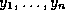at time points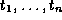which are modelled as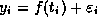, where f is the function to be estimated and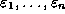i.i.d. Gaussian white Noise with mean 0 and variance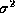. One approach to this problem is wavelet shrinkage, but it has the disadvantage that it requires the number of data points to be a power of two and the time points to be regularly spaced, i.e.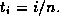In the first chapter we give a short introduction to the thesis and discuss the problem of non-parametric regression as well as the problem with unequally spaced data within the approach of wavelet shrinkage. We review some material on wavelets, the discrete wavelet transform and non-parametric regression in the second chapter.

The third chapter deals with a fast O(n) algorithm for calculating the variances of wavelet coefficients that are obtained by applying the discrete wavelet transform to correlated normal noise with mean 0 and covariance matrix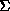, whereis a band matrix. We use this algorithm to introduce a new method for thresholding unequally spaced data in the fourth chapter. We also review contributions of other authors to this topic, present an implementation of the new procedures in the software package WaveThresh which runs under S-Plus and apply the new method to two real data sets.

In the fifth chapter we analyse different threshold selections by calculating the exact mean square error for four test signals and different choices of parameters like different noise levels, hard and soft thresholding rules etc. In particular we analyse versions of Donoho and Johnstone's VisuShrink and SureShrink.

The sixth chapter deals with robust wavelet techniques which perform well even if the the noise is not normally distributed or if the data set is disturbed by several consecutive outliers. Two new algorithms are developed which make use of the new methods for thresholding unequally spaced data and calculating the variances of wavelet coefficients.

The last chapter summarises the results of this thesis and gives the prospects for possible future research.

## Software

Several algorithms were developed in this thesis and implemented in WaveThresh. They can be downloaded as C and S functions along with further S procedures that generate some of the figures. The package needs WaveThresh 3 which is not yet available officially. See the WaveThresh home page for details.

The installation is easy:

• Unpack the archive with gzip oldmakegrid.tar.gz and tar -xf oldmakegrid.tar
• Change the directory: cd makegrid
• Install the software: ./setup